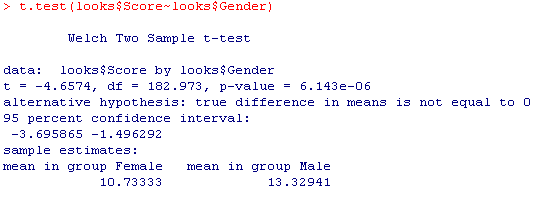## Hypothesis Test for a Difference in Two Population Means (2 of 2)

### Learning Objectives

• Under appropriate conditions, conduct a hypothesis test about a difference between two population means. State a conclusion in context.

On this page, we practice the hypothesis test for a difference in two population means (also called the two-sample t-test).

## Using Technology to Run the Hypothesis Test

When dating someone, what matters more to you: looks or personality? This question was the focus of a community college student’s class project for an introductory statistics course. She devised a 25-point scale. An answer of 1 means “personality matters most and looks don’t matter at all.” A score of 25 means “looks matter most and personality does not matter at all.” Her hypothesis is that the mean scores for males and females will differ, but she does not have an opinion about which population will have a higher mean score.

Here are her hypotheses.

• H0: μ1 – μ2 = 0
• Ha: μ1 – μ2 ≠ 0

We can also write the hypotheses as follows.

• H0: μ1 = μ2
• Ha: μ1 ≠ μ2

She chose a random sample of 10 classes from the schedule at Los Medanos College and distributed surveys in those classes. Survey respondents totaled 239 students: 150 females and 85 males.

We used her data to run a hypothesis test for a difference in two population means.

Here is the relevant output for our example:According to R, the P-value of this test is so small that it is essentially 0. How do we interpret this?

A P-value that is practically 0 means that it would be almost impossible to get data like that observed (or even more extreme) had the null hypothesis been true.

More specifically to our example, if there were no differences between females and males with respect to value they place on looks versus personality, it would be almost impossible (probability approximately 0) to get data where the difference between the sample means of females and males is -2.6 (that difference is 10.73 – 13.33 = -2.6) or higher.

## Identify the P-value

Remember to use the printout of the results in the above example to answer the questions below.